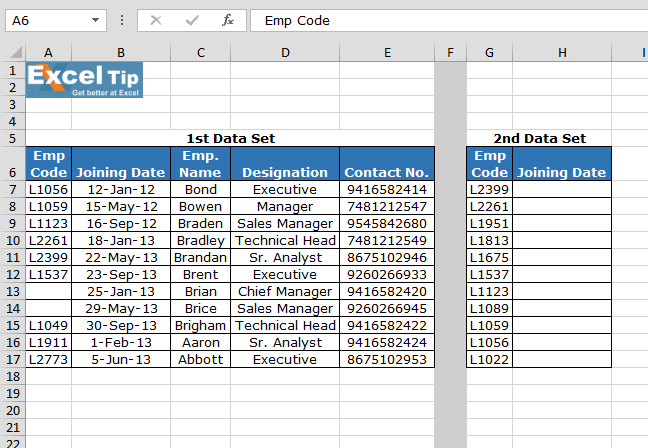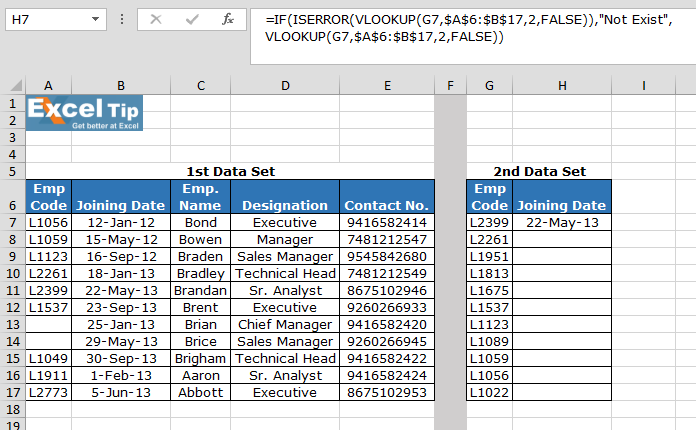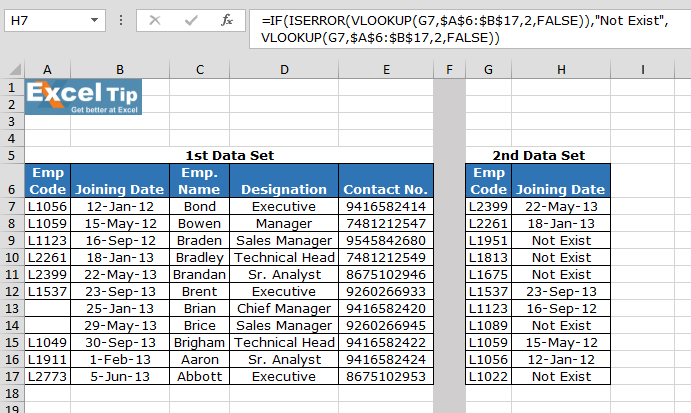Using ISERROR along with VLOOKUP function

In this article, we will learn how to use ISERROR function along with VLOOKUP function to ignore the error as result in Microsoft Excel.

Considering we have this situation where we want to create a formula to check for each successful search. The formula should return that number, when the match is found. And, if an exact match is not found, the formula should return a text message to that effect, rather than an error in Microsoft Excel.

If it is error in Excel Vlookup formula, we will use IF, Iserror and Vlookup function to prevent the #N/A error.

Let’s take an example to understand how we can prevent a Vlookup function from returning an error when an exact match is not found.

Example: We have 2 employees’ data sets. 1st data set in range A2:E13 with contains the details like employee code, date of joining, employee name, designation, contact number. The 2nd data set contains the employee code and the date of joining is to be obtained from the 1st data set• Enter the formula in cell H3
• Press Enter
• The function will return joining date• Copy the same formula in the rangeWe would love to hear from you, do let us know how we can improve, complement or innovate our work and make it better for you. Write us at info@exceltip.com

## Users are saying about us...

1.can you please explain the below formula –>

=IF(ISERROR(VLOOKUP(\$B18,’Profit Projections’!\$A\$11:\$AB\$110,’Profit Projections’!\$AB\$2,FALSE)),0,VLOOKUP(\$B18,’Profit Projections’!\$A\$11:\$AB\$110,’Profit Projections’!\$AB\$2,FALSE))

questions–
what is the formula trying to do?
why is the range defined twice ?

2.WONDERFUL

•Thanks a lot for the appreciation.